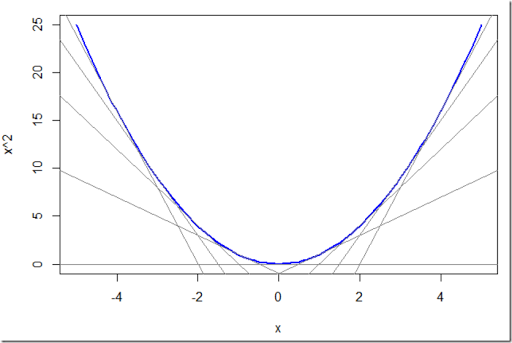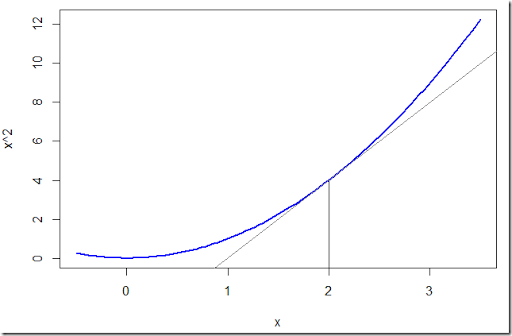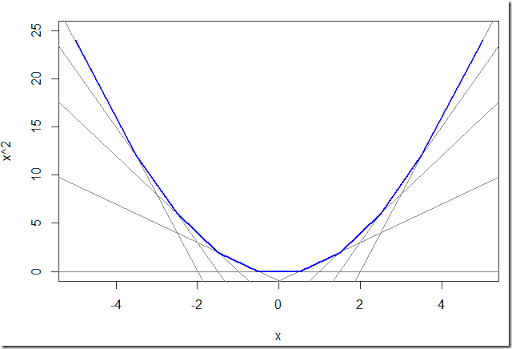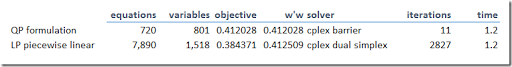## Thursday, April 13, 2017

### QP as LP: piecewise linear functions

I am facing a (convex) QP problem that has some numerical issues for some data sets. Hence as backup I’d like to be able to solve the problem as an LP. One way to do this is using a simple piecewise linear approximation.

Consider the objective function $$\min\> f(x)=x^2$$. Instead of solving this directly we can invent a few linear functions $$y = a_i x + b_i$$ and approximate the problem by:

 \begin{align} \min\>&z\\ &z \ge a_i x + b_i\end{align}

Here is an example how these linear functions can look like:To derive a single linear function we observe the following:

1. Pick a point $$t_i$$, e.g. $$t_i=2$$ in the picture below.
2. We want the function value to match: $$f(t_i) = a_i t_i + b_i$$. I.e. $$a_i t_i + b_i=t_i^2$$.
3. We also want the slope to match: $$\nabla f(t_i) = a_i$$. I.e. $$a_i = 2 t_i$$.This means we can choose:

 \begin{align} &a_i = 2 t_i\\ &b_i = –t_i^2\end{align}

The net effect of this strategy is that we formed a piecewise linear objective that approximates our original quadratic function:Note that there are other linear approximation schemes. This is just a particularly simple one.

##### Example: portfolio optimization

To try this out on a more realistic problem, we consider a standard portfolio optimization problem:

 \bbox[lightcyan,10px,border:3px solid darkblue]{\begin{align} \min \>& \sum_t w_t^2\\ & w_t = \sum_i r’_{i,t} x_i\\ & \sum_i \mu_i x_i \ge R\\ & \sum_i x_i = 1\\ &x_i\ge 0\end{align}}

Here $$r’=r-\mu$$ are the mean adjusted returns.$$R$$ is the minimum expected portfolio return.

In this case our linear model can look like:

 \bbox[lightcyan,10px,border:3px solid darkblue]{\begin{align} \min \>& \sum_t z_t\\ & z_t \ge a_{t,j} w_t + b_{t,j}\>\forall t,j\\ & w_t = \sum_i r’_{i,t} x_i\\ & \sum_i \mu_i x_i \ge R\\ & \sum_i x_i = 1\\ &x_i\ge 0\end{align}}

The $$w_t$$’s vary from $$L_t=\displaystyle\min_i r’_{i,t}$$ to $$U_t=\displaystyle\max_i r’_{i,t}$$. For each $$w_t$$ we choose $$n=10$$ equidistant points between $$[L_t,U_t]$$. We use the FTSE100 dataset from (1), which has:

• number of time periods $$t$$: 717
• number of stocks $$i$$: 83

We see the following results:

• The optimal QP objective is 0.41202816
• The optimal LP objective is 0.37728185

This looks bad, but actually things are better than they appear. When we evaluate $$\displaystyle\sum_i w_t^2$$ for the optimal LP values of $$w_t$$ we have:

• QP objective of LP solution: 0.41246149

Here are the differences in the selected portfolio:

 ----     87 PARAMETER report  QP objective 0.41202816,    LP objective 0.37728185,    w'w from LP  0.41246149 ----     87 PARAMETER xval                   QP          LP stock2   0.11723384  0.10298780stock11  0.13629495  0.14992714stock22  0.06253534  0.06202882stock40  0.09082870  0.10523583stock64  0.08521849  0.08348895stock66  0.17247021  0.17267818stock69  0.00180266stock78  0.09462530  0.09761814stock79  0.05184550  0.04383311stock83  0.18714501  0.18220203
##### Performance

Although the LP model is much larger, it solves in about the same time as the original QP. I see:Note that barrier iterations should really not be compared to simplex iterations. A finer or more coarse grid in the piecewise linear approximation has only affect on the the number of equations in the model (the number of variables will stay the same).

##### References
1. Renato Bruni, Francesco Cesarone, Andrea Scozzari, Fabio Tardella, Real-world datasets for portfolio selection and solutions of some stochastic dominance portfolio models, Data in Brief, Volume 8, September 2016, Pages 858-862
2. QP as LP: cutting planes, http://yetanothermathprogrammingconsultant.blogspot.com/2017/04/qp-as-lp-cutting-planes.html